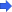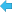Back to Adaptive Vision Studio website

You are here: Start » Filter Reference » Image Features » ImageMoment# ImageMoment

Computes the selected moment of an image in regular and normalized (divided by sum of pixel values) variant.

Name Type DescriptioninImage Image Input imageinRoi Region* Range of pixels to be processedinMomentType ImageMomentTypeinCentral BooloutMoment RealoutNormMoment Real

### Description

The operation computes the mathematical features of an image called moments. Those are sums computed as follows:

\begin{aligned} Moment_{0,0}(Image,Roi) &= \sum_{p \in Roi} Image(p_x,p_y) \cdot 1 \\ Moment_{0,1}(Image,Roi) &= \sum_{p \in Roi} Image(p_x,p_y) \cdot p_y \\ Moment_{1,0}(Image,Roi) &= \sum_{p \in Roi} Image(p_x,p_y) \cdot p_x \\ Moment_{0,2}(Image,Roi) &= \sum_{p \in Roi} Image(p_x,p_y) \cdot p_y^2 \\ Moment_{1,1}(Image,Roi) &= \sum_{p \in Roi} Image(p_x,p_y) \cdot p_x p_y \\ Moment_{2,0}(Image,Roi) &= \sum_{p \in Roi} Image(p_x,p_y) \cdot p_x^2 \end{aligned}

The summing is conducted over all pixels in inRoi region, while $$p_x$$ and $$p_y$$ denote, accordingly, x and y coordinate of a pixel.

When inCentral parameter is set, the image is shifted before computations, so that its mass center is at location (0,0).

### Hardware Acceleration

This operation supports automatic parallelization for multicore and multiprocessor systems.

### Errors

This filter can throw an exception to report error. Read how to deal with errors in Error Handling.

List of possible exceptions:

Error type Description
DomainError Black image on input in ImageMoment.
DomainError Empty region on input in ImageMoment.
DomainError Region exceeds an input image in ImageMoment.

### Complexity Level

This filter is available on Advanced Complexity Level.